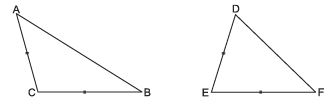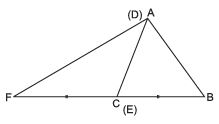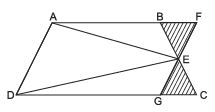Courses

# Hots Questions- Areas of Parallelograms and Triangles Class 9 Notes | EduRev

## Class 9 : Hots Questions- Areas of Parallelograms and Triangles Class 9 Notes | EduRev

The document Hots Questions- Areas of Parallelograms and Triangles Class 9 Notes | EduRev is a part of the Class 9 Course Class 9 Mathematics by VP Classes.
All you need of Class 9 at this link: Class 9

Question 1. If two sides of one triangle are equal to the two sides of another triangle and the contained angles are supplementary; show that the two triangles are equal in area.Let ∆ABC and ∆DEF are such that AC = DE; CB = EF and ∠ABC + ∠DEF = 180°.
Now, allow ∆DEF fall along ∆ABC such that DE falls on AC and B and F are on opposite directions.
∵∠ACB + ∆DEF = 180°⇒ BCF is a straight line
∴ We get a ∆AFB, in which C is mid point of FB.
∴ AC is median of ∆AFB, Median divides the ∆ into two triangles equal in area.
⇒ ar (∆AFC) = ar (∆ABC)
⇒ ar (∆DEF) = ar (∆ABC).

Question 2. In a trapezium ABCD, where AB || DC, E is the mid point of BC. Prove that ∆AED = ½ trap. ABCD.Hint: Through E, draw FEG || AD meeting AB and CD in F and G respectively.
∴∆ BEF ≅ ∆ CEG
⇒ ar trap. ABCD = ar (||gm ADGF)
⇒ ∆ AED = ½ ||gm ADGF

Offer running on EduRev: Apply code STAYHOME200 to get INR 200 off on our premium plan EduRev Infinity!

132 docs

,

,

,

,

,

,

,

,

,

,

,

,

,

,

,

,

,

,

,

,

,

;